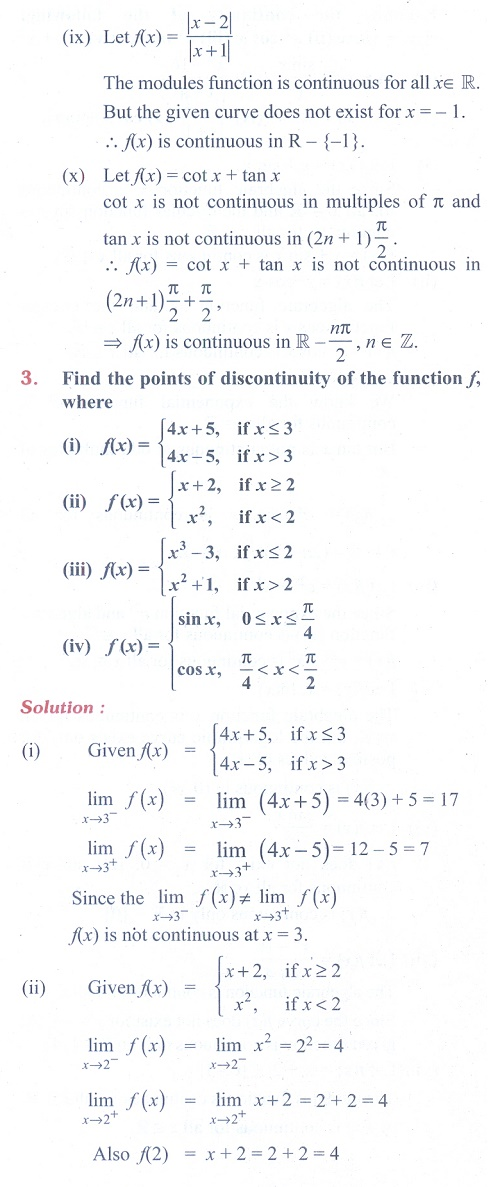Home | | Maths 11th std | Exercise 9.5: Algebra of continuous functions, Removable and Jump Discontinuities

# Exercise 9.5: Algebra of continuous functions, Removable and Jump Discontinuities

Maths Book back answers and solution for Exercise questions - Differential Calculus - Limits and Continuity: Differential Calculus: Algebra of continuous functions, Removable and Jump DiscontinuitiesTags : Differential Calculus | Problem Questions with Answer, Solution | Mathematics , 11th Mathematics : UNIT 9 : Differential Calculus Limits and Continuity
Study Material, Lecturing Notes, Assignment, Reference, Wiki description explanation, brief detail
11th Mathematics : UNIT 9 : Differential Calculus Limits and Continuity : Exercise 9.5: Algebra of continuous functions, Removable and Jump Discontinuities | Differential Calculus | Problem Questions with Answer, Solution | Mathematics